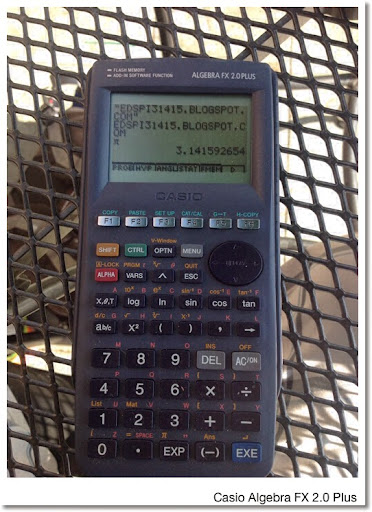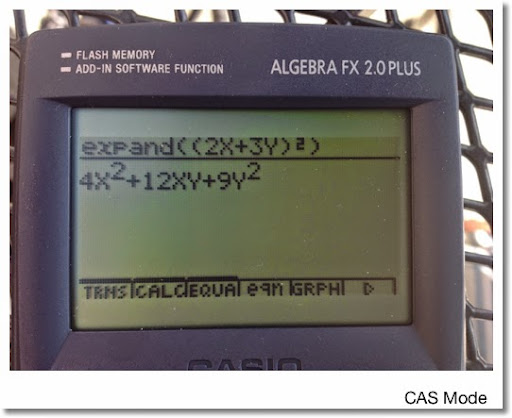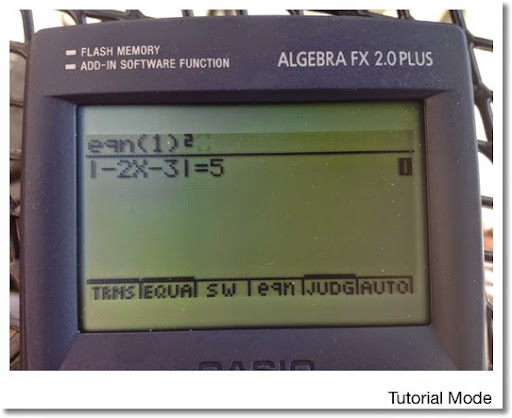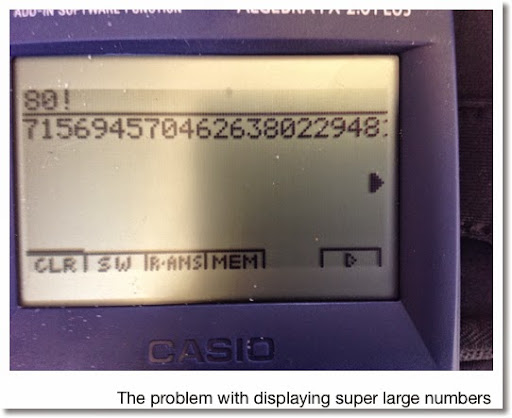## Wednesday, May 28, 2014

### Retro-Review: Casio Algebra FX 2.0 PlusRetro-Review: Casio Algebra FX 2.0 Plus

Production Period: 1999 - 2001 (thank you www.rskey.org)
Memory: 146,000 bytes with 768,000 bytes flash

On the surface, the Casio Algebra FX 2.0 Plus looks like a typical modern Casio graphing calculator: the MENU key displays an iconic menu, the top row is a set of six soft keys, the layout of the keys are similar, and its programming language is the same as its fellow graphic calculators.

I am just going to go over what separates the Algebra FX 2.0 Plus from the other Casio calculators. We can assume it can handle what ever the fx-9860g series (including the Prizm, sans color) does.

What separates the Algebra FX 2.0 from the other Casio graphing calcualtors? First, complex numbers are not only allowed as arguments in transcendental functions (logarithmic and trigonometric functions). Complex numbers are accepted as coefficient in the polynomial and simultaneous equation solvers. Second, instead of the usual 6, the polynomial and simultaneous equations solvers work orders up to 30. The big difference, is that calculator has CAS (computer algebraic system) capabilities.

Being Casio, the CAS functionality is accessed in a separate mode. Make that three separate modes.

With each CAS mode allowing equations, algebraic expressions, integers, and fractions to be expanded, factored, simplified, and approximated; the mode dictated which other options were available:

CAS Mode: This had the full suite of CAS functions on the Algebra FX 2.0 Plus: derivative, integral, sums, the ability to work with ∞, extracting parts of an expression, calculating arc length, tangent lines, GCD, and LCM. (I think I got most of it, it not all.)

Algebra Mode: This is a limited CAS mode, probably aimed at younger students. Only x!, |x|, denominator and numerator extraction, LCM, and GCD are available. Not sure what the Algebra mode exclusive arrange and replace commands do.

Tutorial Mode: Choose from a library of 45 equation types and use the CAS commands to solve them. What commands are available is dependent on the type of equation being solved.

Something I thought was strange with the CAS mode was that it could handle numbers bigger than 10^99. Try to get the approximation of that number, you get an overflow error. And really large numbers have digits cut off in the display so reading them presented a challenge.

For example: 80! (80! ≈ 7.1569 × 10^118)

Display: 7156945704626380229481(?) 6945704626380229481153(?)...

Trying approx(80!) returns an overflow error.

A impressive mode was the Differential Equation mode, where you can get a slope field plot of various types of equations including:
* 1st Order Linear
* 1st Order Bernoulli
* 1st Order Exact
* 2nd Order Linear and higher
* 1st Order Linear Systems

I bought my Algebra FX 2.0 I think more than a decade ago on clearance. I think this calculator was underrated due to its short production life. Was the Algebra FX 2.0 a predecessor to the ClassPad Series? Seems like it.

That is my retro-view. Hope you liked it. Talk to you next time!

Eddie

This blog is property of Edward Shore. 2014

### Casio fx-CG50: Sparse Matrix Builder

Casio fx-CG50: Sparse Matrix Builder Introduction The programs can create a sparse matrix, a matrix where most of the entries have zero valu...